### Home > GC > Chapter 6 > Lesson 6.2.1 > Problem6-43

6-43.
1. Use your triangle tools to solve for x in the triangles below. Homework Help ✎

1.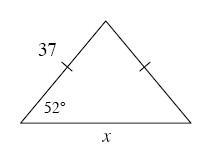2.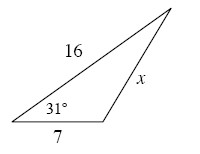3.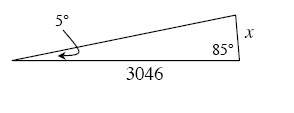4.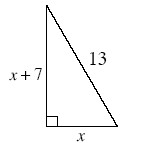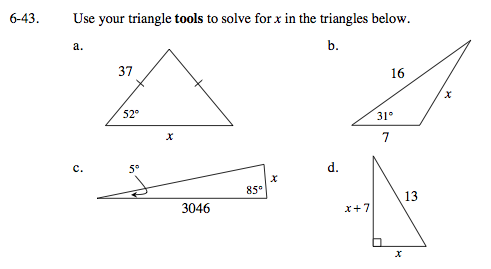What do the tick marks on the sides of the triangle mean?
What do they tell you about the lengths of the sides and the measures of the angles?

Use the Law of Sines to find x.

x ≈ 45.56

Use the Law of Cosines to solve for x.

x ≈ 10.63

Find the size of the missing angle.

Use the sine ratio.

x = 265.48

What special right triangle has a hypotenuse of 13?